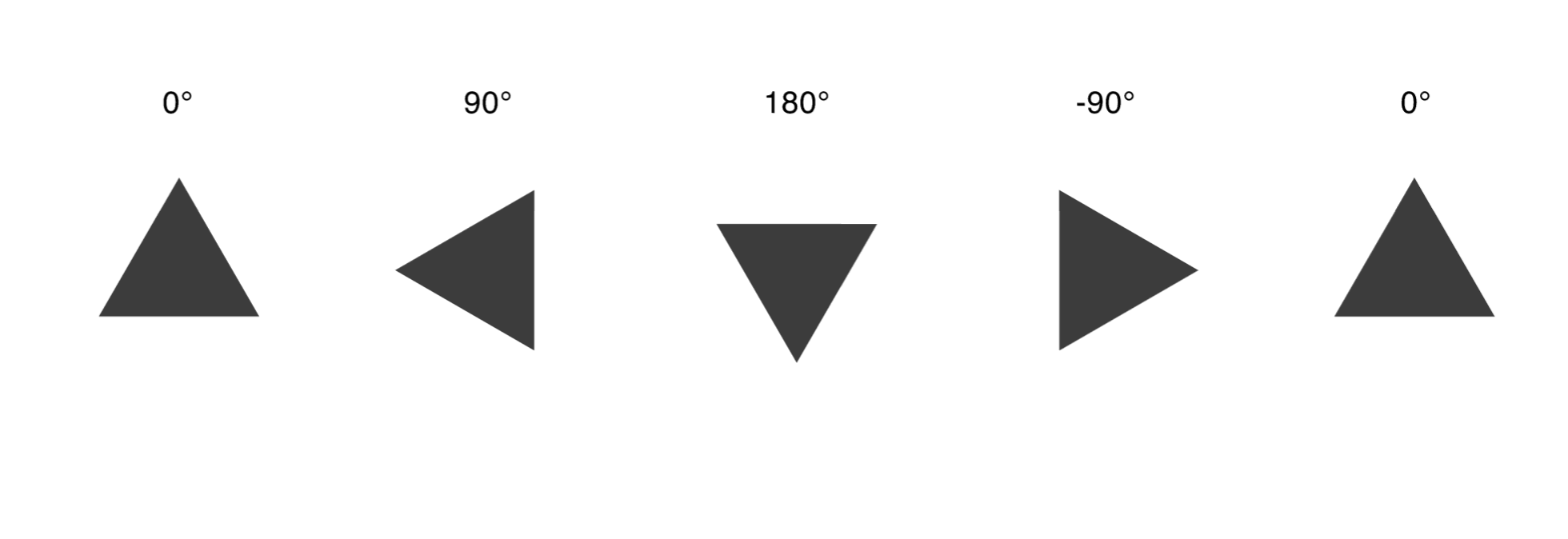# Figma rotationFigma rotation from figma plugin docs

## [Note] The rotation value needs to be inverted on the client side.​

to rotate something to the clockwise direction,

• figma: -n
• client: +n (css)

the transform origin

• figma: not specified (always top left)
• client: top left

so the conversion from figma to css will be like below

``# figma{  x: 100,  y: 100,  rotation: 10,}# css.rotate-n {  top: 100;  left: 100;  transform: rotate(-10deg);  transform-origin: top left;}``

## Transform?​

While figma and other major design tools has both transform value, and explicit rotation value (which can be calculated from transform value), The intuitive way to represent a rotation value is by using a `Rotation` token. Overall all figma node properties, the only two property that has impact to final transform (based on css) is `scale` and `rotation`.

But those two value comes from different property, one from `node#roation` (or `node#relativeTransform`), one from `node#constraint#scale` - a dynamic `scale` representor.

For this reason, while we tokenize the design, we use `Rotation` token rather than `Transform` token.

e.g.

``// node example (this is a abstract example, the syntax may differ.)// [scale only example]{    rotation: 0,    constraints: "SCALE"}// in this case, only scale property will be assigned to final transform value.// Step 1 tokenizationScale(  scale: aspect_ratio, // a dynamically calculated value to make scale responsive  child: node)// Step 2 merge transformTransform(  scale: matrix4, // a scale value that is represented as matrix 4  child: node)// ------------------------------------------// [rotation only example]{    rotation: 30,    constraints: "MIN"}// in this case, only scale property will be assigned to final transform value.// Step 1 tokenizationRotation(  rotation: 30,  child: node)// Step 2 merge transformTransform(  rotation: 30,  child: node)// ------------------------------------------// [rotation + scale example]{    rotation: 30,    constraints: "SCALE"}// Step 1 tokenizationTransforms(    transforms: [    Rotation(      rotation: 30    ),    Scale(        scale: aspect_ratio, // a dynamically calculated value to make scale responsive      )  ]    child: node)// Step 2 merge transformTransform(  rotation: 30,  scale: matrix4,  child: node)``

## Web - css​

rotate vs rotateZ vs rotate3d

The difference between the three functions is whether 3D is supported or not and whether only z is expressed. The code below shows the same result when used only flat.

``transform: rotate(10deg)/* equal */transform: rotateZ(10deg)/* equal */transform: rotate3d(0, 0, 1, 10deg)``

All three can produce the same result, but 2d `rotate` is used because 3d rotate and 2d rotate must be separated.

Also `rotate` and `rotateZ` can be used in the same Terms. Meanwhile we only support `rotate`, which has more compatibility with various browsers.

## Flutter​

RotateBox vs Transform.rotate

`RotateBox` receives a turn with a value represented with Matrix4, so it is not suitable to express various degree values. Meanwhile we only support `Transform.rotate` since `Transform.rotate` can be represented with single value - `rotation`

how to set degree

`degrees * math.pi / 180`

RotatedBox

``RotatedBox(    quarterTurns: 0,    child: child);``

Transform.rotate

``Transform.rotate(    angle: 90 * math.pi / 180,    child: child);``

## Rotation as Animated value​

Rotation Animation is a working draft - the flag & code gen is not supported.

The goal of `design to code` is to transform the design itself into code so that users can use it right away without any chores. There are two main cases where most rotations are used. One is when a fixed form is rotated from multiple angles, and the other is animation.

Considering the case where the user rotates the corresponding figure for animation, it is an animation, but it is better in terms of usability to provide it as a stopped animation. Therefore, we provide it as a frozen animation.

``// you can set this via setting a animation flag// if animated, rotation value rather than 0 will automatically interpreted as rotation transitionnode.name = "--animated";// if `animated-rotation` flag is givven, we will always interpret the rotation as animated value (even if it is 0)node.name = "--animated-rotation";``

Flutter#RotationTransition (For animation)

``// WIP``

Css / js - rotate animation

``/* WIP */``

## Note for assistant​

On assistant, on a plugin version, the assets are exported as-is including the rotated snapshot. which means having rotation on already rotated image will cause incorrect visualization. i.e having 30 rotated triangle vector, the graphics exports as-is, in total 60 rotated visually.